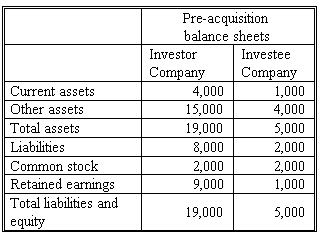### CFA Practice Question

There are 334 practice questions for this study session.

### CFA Practice QuestionIf Investor Company, by issuing new stocks, acquires a 30% interest in Investee Company at book value, under the proportionate consolidation method, Investor Company will report total assets of

A. \$20,500.
B. \$19,000.
C. \$24,000.

Under the proportionate consolidation method, the percentage owned of the Investee Company assets and liabilities are added to the Investor Company's balance sheet. In this case, total assets will be \$19,000 + 30% * \$5,000 = \$20,500.

User Comment
robbe1 If the stake is bought with cash, total assets stays at \$19k.
turtle exactly :-)
raner why? will the CA(Cur Asset) account be reduced by the cash paid out?
ilzina if investor company obtains additional funds by issuing new shares, the assets of investor go up by 1k. so 20k -1k (payment) + 30% x5k = 20.5k
rodney176 The question did mention its an all stock transaction
davidt876 not at all robbe and turtle... when you purchase with cash, you gain the assets of the investee less the cost of acquisition. and because A=L+E, the assets acquired (A) will always be greater than the cost of the company' equity (E) unless Liabilities=0. so the net effect of a cash purchase is usually positive on assets.

using this question, a cash purchase would be:
cost = 0.3*BV of investee's equity = 0.3*(2,000+1,000) = 900

Current assets (cash) = 4000 + (0.3*1,000) - 900(cost) = 3,400
Other assets = 15,000 + (0.3*4,000) = 16,200
Total Assets = 19,600

Liabilities = 8,000 + (0.3*2,000) = 8,600
Common stock = 2,000 + (0.3*(2,000+1,000)) = 2,900
Retained earnings = 9,000 - 900(cost) = 8,100
Total liabilities & equity = 19,600

the 900 cost of acquisition is fed through the income statement to reduce equity via retained earnings - this is to balance out the decrease in assets when the investor paid out cash [A(-900) = L + E(-900)] and then everything else is just added at the % share